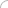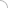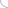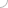# Hardware and Software Design interview questions and answers

## Hardware and Software Design interview questions and answers

### 1. Explain finite automata? 2. What do you understand by a Turing machine? 3. What do you understand by parsing? 4. What are the different models of computation? 5. What are embedded systems? 6. What are the common design metrics? 7. What do you understand by design technology? 8. Explain the design productivity gap? 9. What are the steps involved in optimizing single-purpose processors? 10. What do you understand by an operation? 11. What is the various design tools used nowadays? 12. What is the purpose of debugging? 13. What are ASIP`s? 14. What is UART? 15. What are common memory types? 16. What is the purpose of the MMU? 17. Explain microprocessor interfacing. 18. What is arbitration? 19. Explain daisy-chain arbitration? 20. Give a brief description of multi level bus architectures? 21. What is the CAN bus? 22. Name the common wireless protocols? 23. What are the different areas of application of hardware and software design? 24. What is a priori in design? 25. What are the disadvantages of defining a priori in design? 26. What is POLIS? 27. Explain with the help of an example a CFSM. 28. What is the design flow implemented in POLIS? 29. What is VHDL? 30. What are the differences between Logical design and physical design? 31. Name a few alternative design methodologies. 32. What do you understand by RAD? 33. What do you understand by JAD? 34. Explain state charts? 35. What are the advantages of state charts? 36. Explain the tree of states of state chart? 37. Describe the process of conversion of StateChart to an FSM? 38. What are the different models of computation? 39. Explain the Kahn Process network? 40. Explain synchronous data flow in terms of design? 41. What do you understand by clustering? 42. What is the closeness function? 43. What is hierarchical clustering? 44. What do you understand by HW/SW partitioning? 45. What are pareto points? 46. What are evolutionary algorithms? 47. Explain what you understand by solution space? 48. What is simulating time? 49. How is simulation time tracked? 50. What do you understand by WCET? (adsbygoogle = window.adsbygoogle || []).push({}); Write your comment - Share Knowledge and Experience (adsbygoogle = window.adsbygoogle || []).push({}); (adsbygoogle = window.adsbygoogle || []).push({});Interview questionsLatest MCQs » General awareness - Banking » ASP.NET » PL/SQL » Mechanical Engineering » IAS Prelims GS » Java » Programming Language » Electrical Engineering » English » C++ » Software Engineering » Electronic Engineering » Quantitative Aptitude » Oracle » English » Finance Home | About us | Sitemap | Contact us | We are hiring © Copyright 2016. All Rights Reserved. Terms of use  |  Follow us on Facebook!